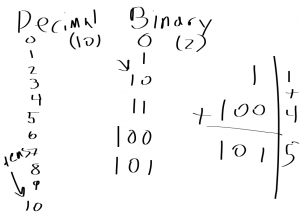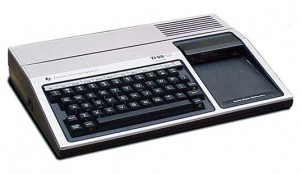# Homeschool Technology Lesson 2: Binary (Continued)

The second homeschool technology lesson was largely a reminder of the first – I reviewed binary. I wrote on the “board” (iPad connected to a TV) decimal numerals…

## Homeschool TechnologyThen for binary, base-2, the first column goes up to… 2 minus 1: 1. Then add a columnI explained again, slowly and repeatedly that decimal, which is base-10, the first column goes up to 10 minus 1: 9. Then you have to add a column. Repeat that over
and over.

Q: For base-5, what does the first column go up to?

A: 4

When you add that second column in base-10, it’s worth ten. So when you add the second column in base-2, it’s worth 2! You got it! So this is lesson 10 (binary)!

Q: For base-8, what is the second column worth?

A: 8

Each column in base-10 from there is ten multiplied by the previous column. So the second column is 10 times the first column number (1): 1×10=10.

The third column is 10 times the value of the second column (10) so 10×10=100.

1×10=10, 10×10=100, 100×10=1000, etc.

10 – 100 – 1000 – 10000, etc.

Same for base-2 – multiply by 2: 2, 4, 8, 16, 32, 64, 128, 256, 512, 1024, 2048, etc.

1 (one), 10 (two), 100 (four), 1000 (eight), 10000 (sixteen), 100000 (thirty-two), etc.

Then we did some addition…

1 (binary, one) plus 100 (binary, four) is 101 (five).

Counting in binary goes:

0 (zero)

1 (one)

10 (add column which is two)

11 (three)

100 (add column, 2×2=4 decimal)

etc.

That was the homeschool technology lesson 2 for more binary. I want to really drill this foundation home before moving on. Once we have this down with review and practice, I’ll probably move on to hexadecimal and ASCII. The on to how computers store files and such.

Side note: When I was first learning computer science, I learned from reading manuals thatcame with my TI-99/4A with Extended BASIC. I’d read about a BASIC statement (e.g., if-then) and then write something using it.

But then (and still) I learned a lot from reading so I didn’t know how to pronounced things. I thoughts ASCII (pronounced like rhyming “tasky”) was pronounced like A-S-C-2. I thought the I’s were Roman numeral 2.